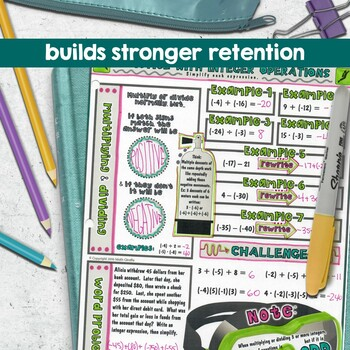# Integer Operations Doodle Notes6th - 7th
Subjects
Standards
Resource Type
Formats Included
• PDF
Pages
2 plus answer keys, alternate options, & info

### Description

Operations with Negative Numbers (Integers): "doodle notes" -

When students color or doodle in math class, it activates both hemispheres of the brain at the same time. There are proven benefits of this cross-lateral brain activity:

- new learning

- relaxation (less math anxiety)

- visual connections

- better memory & retention of the content!

Students fill in the sheets, answer the questions, and color, doodle or embellish. Then, they can use it as a study guide later on.

Content includes:

- subtracting integers

- multiplying and dividing integers

- concepts & real-world application

- examples and practice

- operations with 3 or more numbers (positive and negative)

Visual note taking strategies like sketch notes or doodle notes are based on dual coding theory.  When we can blend the text input with graphic/visual input, the student brain processes the information differently and can more easily convert the new learning into long-term memory.

This strategy also integrates the left and right hemispheres of the brain to increase focus, learning, and retention!

You might also like:

Middle School Pre-Algebra Super Bundle

Consumer Math & Personal Finance: Complete Unit

Conquest Game - Algebra Set 1 - Solving Equations

Total Pages
2 plus answer keys, alternate options, & info
Included
Teaching Duration
45 minutes
Report this Resource to TpT
Reported resources will be reviewed by our team. Report this resource to let us know if this resource violates TpT’s content guidelines.

### Standards

to see state-specific standards (only available in the US).
Apply and extend previous understandings of addition and subtraction to add and subtract rational numbers; represent addition and subtraction on a horizontal or vertical number line diagram.
Understand subtraction of rational numbers as adding the additive inverse, 𝘱 – 𝘲 = 𝘱 + (–𝘲). Show that the distance between two rational numbers on the number line is the absolute value of their difference, and apply this principle in real-world contexts.
Apply and extend previous understandings of multiplication and division and of fractions to multiply and divide rational numbers.
Apply properties of operations as strategies to multiply and divide rational numbers.
Solve real-world and mathematical problems involving the four operations with rational numbers.